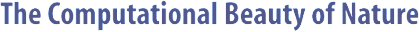Computer Explorations of Fractals, Chaos,Complex Systems, and Adaptation· title page · home* · cover artwork · jacket text · table of contents · the author* · ordering informationBook Contents
 · three themes · part synopses · selected excerpts · all figures from book · quotes from book · glossary from book · bibliography · slide showSource Code
 · overview &documentation · FAQ list* · download source code · java appletsMiscellany
 · news* · reviews & awards · errata · for educators · bibliography (BibTeX format) · other linksJULIA Documentation

```

```

#### NAME

```       julia - make a plot a Julia set

```

#### SYNOPSIS

```       julia -help
or
julia  [-width integer] [-height integer] [-maxit integer]
[-levels  integer]  [-bail  double]  [-ulx  double]
[-uly  double] [-lly double] [-cr double] [-ci dou-
ble] [-box integer] [-bulx double]  [-buly  double]
[-blly  double] [-idiv integer] [-rev] [-inv] [-mag
integer] [-term string]

```

#### DESCRIPTION

```       A Julia set is drawn according to  the  specified  parame-
ters.   The  image  is  computed  by iterating the complex
equation z(t) = (z(t-1))^2 + c, where c = (cr + ci) is the
complex  point  that  determines  which Julia set is being
computed and z = (x + yi) corresponds to an (x, y)  screen
coordinate  with  the  initial  value of z(0) = 0.  If the
system diverges at time k (i.e., |z(k)|  >  BAIL)  then  a
point  at  (x, y) is plotted with the grayscale color (k /
IDIV + (k % IDIV) * (LEVELS  /  IDIV))  %  LEVELS),  which
reduces to (k % LEVELS) with an IDIV of 1.

```

#### OPTIONS

```       -width integer
Width of the plot in pixels.

-height integer
Height of the plot in pixels.

-maxit integer
Maximum number of iterations before automatic bail-
out.

-levels integer
Number of plot (gray) levels to use.

-bail double
Value of |z| to end iteration,  i.e.,  the  bailout
value.

-ulx double
Upper-left corner x-coordinate.

-uly double
Upper-left corner y-coordinate.

-lly double
Lower-left corner y-coordinate.

-cr double
Real component of c.

-ci double
imaginary component of c.

-box integer
Line width for a box.  If zero, no box is drawn.

-bulx double
Box's upper-left x-coordinate.

-buly double
Box's upper-left y-coordinate.

-blly double
Box's lower-left y-coordinate.

-idiv integer
Iteration  divisor.    When  greater than one, this
creates a banding effect.

-rev   Reverse all colors but first?

-inv   Invert all colors?

-mag integer
Magnification factor.

-term string
how to plot points

```

#### COLORS

```       The four permutations of using or not using -rev and  -inv
will  yield  four  different coloring schemes.  Try it and
see.

```

#### MISCELLANY

```       The method for  choosing  the  viewable  region  may  seem
counter-intuitive  at  first, but it has some nice proper-
ties.  In particular, selecting the exact (x,  y)  coordi-
nates  for  the  upper-left  corner and only selecting the
lower right y coordinate forces both the x and y scales to
be  identical  since all scales are uniquely determined by
these values along with the plot width and height.  If you
then  change the width or height of the plot, the relative
scales will still match up.  The options for making a  box
work similarly.

```

#### BUGS

```       No  sanity  checks  are performed to make sure that any of
the options make sense.  In particular, the bounding  cor-
ners  can  be  screwed  up, and division by zero can occur
with a malicious setting of IDIV.

```

#### AUTHOR

```       Copyright (c) 1997, Gary William Flake.

Permission granted for any use according to  the  standard
GNU ``copyleft'' agreement provided that the author's com-
ments are neither modified nor removed.   No  warranty  is
given or implied.

```Copyright © Gary William Flake, 1998-2002. All Rights Reserved.Last modified: 30 Nov 2002